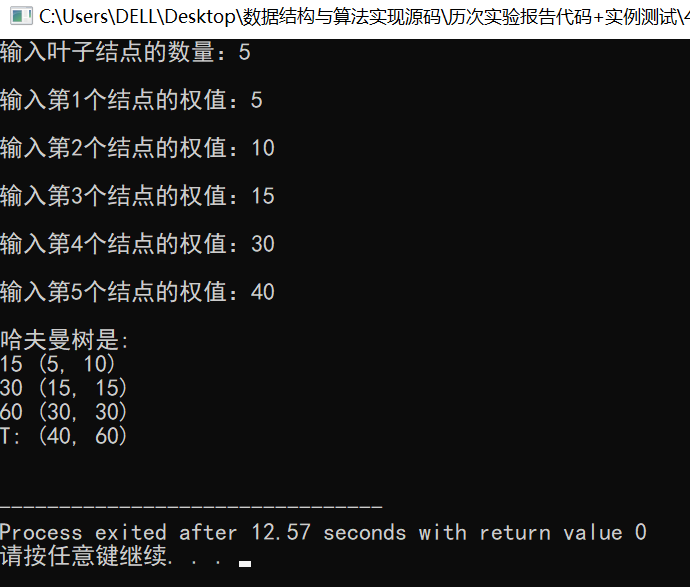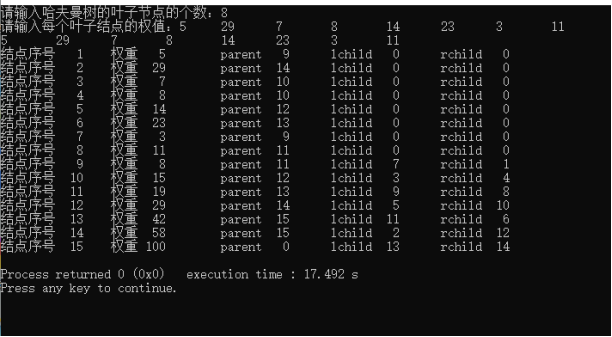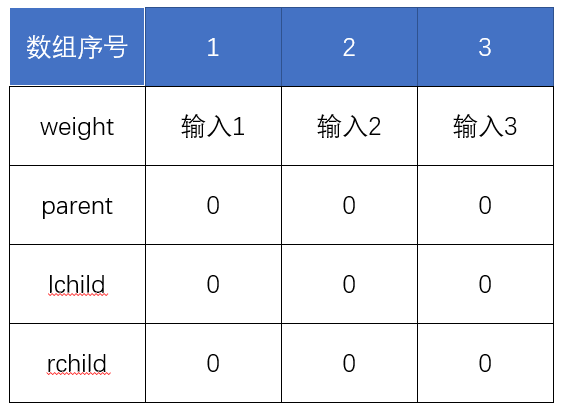• HuffmanTree.h文件： 哈夫曼树存储结构与创建函数 HuffmanCreatTest.cpp文件： 用于测试 效果图：（如下） 效果图： HuffmanTree.h文件： #include <stdio.h> #include <stdlib.h> typedef struct...

本文包含两个文件的代码和一张测试效果图：

• HuffmanTree.h文件： 哈夫曼树的存储结构与创建函数
• HuffmanCreatTest.cpp文件： 用于测试
• 效果图：（如下）

效果图：HuffmanTree.h文件：

#include <stdio.h>
#include <stdlib.h>

typedef struct{
int weight;
int parent,lchild,rchild;
}HTNode, *HuffmanTree;

void Select(HuffmanTree HT, int n, int *s1, int *s2)
{
int minum;      // 定义一个临时变量保存最小值?
for(int i = 1; i <= n; i++)     // 以下是找到第一个最小值
{
if(HT[i].parent == 0)
{
minum = i;
break;
}
}
for(int i = 1; i <= n; i++)
{
if(HT[i].parent == 0)
if(HT[i].weight < HT[minum].weight)
minum = i;
}
*s1 = minum;
// 以下是找到第二个最小值，且与第一个不同
for(int i = 1; i <= n; i++)
{
if(HT[i].parent == 0 && i != *s1)
{
minum = i;
break;
}
}
for(int i = 1; i <= n; i++)
{
if(HT[i].parent == 0 && i != *s1)
if(HT[i].weight < HT[minum].weight)
minum = i;
}
*s2 = minum;
}

void CreateHuffmanTree(HuffmanTree &HT, int *w, int n)
{
if(n <= 1){
return;
}
int m, i, s1, s2;
m = 2 * n - 1;
HT = (HuffmanTree)malloc((m+1)*sizeof(HTNode)); // 分配空间
for(i = 1; i <= m; i++){ //将1~m号结点初始化
HT[i].weight = w[i];
HT[i].parent = 0;
HT[i].lchild = 0;
HT[i].rchild = 0;
}
printf("\n哈夫曼树是: \n");
int num = 1;
for(i = n + 1; i <= m; i++){ //通过n-1次的【选择】、【删除】、【合并】来创建哈夫曼树

//【选择】在HT[k](1<=k<=i-1)中选择两个：双亲域为0且权值最小的结点，并返回它们在HT中的序号s1和s2
Select(HT, i-1, &s1, &s2);

//【删除】得到新结点i，从森林中删除s1，s2，即将s1和s2的双亲域由0改为i
HT[s1].parent = i;
HT[s2].parent = i;

// 【合并】将s1和s2的权值和作为一个新结点的权值依次存入到数组的第n+1之后的单元中，同时记录这个新结点左孩子的下标s1，右孩子的下标为s2
HT[i].lchild = s1;
HT[i].rchild = s2;
HT[i].weight = HT[s1].weight + HT[s2].weight;
if(i <= m - 1){
printf("%d (%d, %d)\n", HT[i].weight, HT[s1].weight, HT[s2].weight);
}
else{
printf("T: (%d, %d)\n", HT[s1].weight, HT[s2].weight);
}
}
printf("\n");
}

HuffmanCreatTest.cpp文件：

#include "HuffmanTree.h"

int main()
{
HuffmanTree HT;
int n;
printf("输入叶子结点的数量：");
scanf("%d", &n);
int w[n+1];

for(int i=1; i<=n; i++)
{
printf("\n输入第%d个结点的权值：", i);
scanf("%d", &w[i]);
}
CreateHuffmanTree(HT, w, n);
}

展开全文数据结构
• 哈夫曼树——顺序存储

千次阅读 2019-11-16 17:56:13
1、创建一棵哈夫曼树，顺序存储方式 2、可键盘输入叶子结点的权值 3、输出各节点的权值、父母域、左右孩子域 代码 #include<stdio.h> #include<stdlib.h> typedef struct { int weight; int parent,...

内容

1、创建一棵哈夫曼树，顺序存储方式
2、可键盘输入叶子结点的权值
3、输出各节点的权值、父母域、左右孩子域

代码

#include<stdio.h>
#include<stdlib.h>
typedef struct
{
int weight;
int parent,lchild,rchild;
}HTNode,*HuffmanTree;

void CreateHuffmanTree(HuffmanTree *HT,int n);
int Select(HuffmanTree *HT,int i);

int main()
{
int i;
HuffmanTree HT;
int n;
printf("请输入哈夫曼树的叶子节点的个数：");
scanf("%d",&n);
CreateHuffmanTree(&HT,n);
for(i=1;i<2*n;i++)		//输出各节点的权值、父母域、左右孩子域
{
printf("结点序号%4d\t权重%4d\tparent%4d\tlchild%4d\trchild%4d\t\n",i,HT[i].weight,HT[i].parent,HT[i].lchild,HT[i].rchild);
}
return 0;
}

/*函数：void CreateHuffmanTree(HuffmanTree *HT,int n)
作用：构造一棵哈夫曼树
输入：HuffmanTree数组的首地址和叶子结点的个数n*/
void CreateHuffmanTree(HuffmanTree *HT,int n)
{
HuffmanTree *T;
int m;
int i;
int s1,s2;
if(n<=1)
return;
m=2*n-1;
*HT=(HuffmanTree)malloc(sizeof(HTNode)*(m+1));       //0号单元未用，所以需要动态分配m+1个单元，HT[m]表示根结点
T=HT;
for(i=1;i<=m;++i)       //将1~m号单元中的双亲、左孩子、右孩子的下标都初始化为0
{
(*HT)[i].parent=0;
(*HT)[i].lchild=0;
(*HT)[i].rchild=0;
}
printf("请输入每个叶子结点的权值：");
for(i=1;i<=n;i++)       //输入前n个单元中叶子结点的权值
{
scanf("%d",&(*HT)[i].weight);
/*--------------------------初始化工作结束，下面开始创建哈夫曼树---------------------------------------*/
printf("%d\t",(*HT)[i].weight);
}
printf("\n");
for(i=n+1;i<=m;++i)
{//通过n-1次的选择、删除、合并来创建哈夫曼树
s1=Select(T,i-1);
(*HT)[s1].parent=i;
s2=Select(T,i-1);
(*HT)[s2].parent=i;   //得到新结点i，从森林中删除s1、s2，将s1、s2的双亲域由0改为i
(*HT)[i].lchild=s1;
(*HT)[i].rchild=s2;   //s1、s2分别作为i的左右孩子
(*HT)[i].weight=(*HT)[s1].weight+(*HT)[s2].weight;     //i的权值为左右孩子权值之和
}
}

/*函数：int Select(HuffmanTree *HT,int i)
作用：在[k](1<=k<=i)中选择两个其双亲域为0且权值最小的结点，并返回他们的在HT中的序号的地址，s1,s2
输入：HuffmanTree数组的首地址和在[k](1<=k<=i-1)中选择两个其双亲域为0且权值最小的结点，并返回他们的在HT中的序号*/
int Select(HuffmanTree *HT,int i)
{
int k,s;
int MIN=100;
for(k=1;k<=i;++k)
{
if((*HT)[k].parent==0)
{
if((*HT)[k].weight<MIN)
{
MIN=(*HT)[k].weight;            //找出权重最小的
s=k;                      //s存放他的序号
}
}
}
return s;
}

输出展开全文C语言 数据结构 哈夫曼树
• 哈夫曼树的构造： 存储结构：线性表、线性存储结构 实现方法：createHuffmanTree import java.util.*; public class HuffmanTreeDemo { public static void main(String[] args) { /* 哈夫曼树的构造： ...

哈夫曼树的构造：

存储结构：线性表、线性存储结构
实现方法：createHuffmanTreeimport java.util.*;

public class HuffmanTreeDemo
{
public static void main(String[] args)
{
/*
哈夫曼树的构造：
存储结构：线性表、线性存储结构
实现方法：createHuffmanTree
*/
Scanner scan = new Scanner(System.in);
int n = Integer.parseInt(scan.nextLine());

HTNode[] HT = createHuffmanTree(scan, n);

scan.close();
}

//创建哈夫曼树，输入：键盘输入、哈夫曼节点数量；   输出：节点组成的哈夫曼数组
public static HTNode[] createHuffmanTree(Scanner scan, int n){
if(n<=1)
return null;

//哈夫曼树初始化
int m = 2*n-1;						//共2n-1个节点
HTNode[] HT = new HTNode[m+1];	    //不用0号单元
for(int i=1; i<=m; i++){
HT[i] = new HTNode();
}
for(int i=1; i<=n; i++){
HT[i].weight = Integer.parseInt(scan.nextLine());
}

//通过n-1次选择、删除、合并操作来创建赫夫曼树
for(int i=n+1; i<=m; ++i){
//在HT(1~i-1)中选择两个双亲为0且权值最小的两个节点，并返回节点序号
int[] s = select(HT, i-1);

HT[i].lchild = s;
HT[i].rchild = s;

HT[i].weight = HT[s].weight + HT[s].weight;

HT[s].parent = i;
HT[s].parent = i;
}

return HT;
}

//选择函数，选择HT树中未被利用过的最小两个点的序号
public static int[] select(HTNode[] HT, int n){
int[] s = new int;

int m1 = 10000;
int m2 = 10000;

for(int i=1; i<=n; i++){
if(HT[i].parent!=0){}

else{
if(m1>HT[i].weight){
m2 = m1;
s = i-1;
m1 = HT[i].weight;
s = i;
}else if(m2>HT[i].weight){
m2 = HT[i].weight;
s = i;
}
}
}

return s;
}
}

class HTNode
{
int weight;
int parent;
int lchild;
int rchild;
public HTNode(){
this.weight = 0;
this.parent = 0;
this.lchild = 0;
this.rchild = 0;
}
}

展开全文数据结构
• 天天都是一个出发点，每天都有一点提高，...《数据结构》课程设计报告设计题目 哈 夫 曼 (Huffman) 编/译 码 器学院名称 信 息 工 程 学 院专 业 班 级 12 计 本 2姓 名 张 翠 翠学 号 1212210217 ______题目：哈...

天天都是一个出发点，每天都有一点提高，每天都有一点收成！

《数据结构》

课程设计报告

设计题目 哈 夫 曼 (Huffman) 编/译 码 器

学院名称 信 息 工 程 学 院

专 业 班 级 12 计 本 2

姓 名 张 翠 翠

学 号 1212210217 ______

题目：哈夫曼(Huffman)编/译码器

一、问题描述

利用哈夫曼编码进行通信可以大大提高信道利用率

缩短信息传输时间

降低传输成本

但是

这要求在发送端通过一个编码系统对待传数据预先编码

在接收端将传来的数据进行译码(复原)

对于双工信道(即可以双向传输信息的信道)

每端都需要一个完整的编/译码系统

试为这样的信息收发站写一个哈夫曼码的编/译码系统

二、设计目标

帮助学生熟练掌握树的应用和基本操作

重点掌握二叉树的存储

这里以哈夫曼树为设计目标进一步提高学生的设计能力及对树的理解

三、任务要求

一个完整的系统应具有以下功能：

1) I：初始化(Initialization)

从终端读入字符集大小n

以及n个字符和n个权值

建立哈夫曼树

并将它存于文件hfmTree中

2) E：编码(Encoding)

利用以建好的哈夫曼树(如不在内存

则从文件hfmTree中读入)

对文件ToBeTran中的正文进行编码

然后将结果存入文件CodeFile中

3) D：译码(Decoding)

利用已建好的哈夫曼树将文件CodeFile中的代码进行译码

结果存入文件TextFile中

4) P：印代码文件(Print)

将文件CodeFile以紧凑格式显示在终端上

每行50个代码

同时将此字符形式的编码文件写入文件CodePrin中

5) T：印哈夫曼树(Tree Printing)

将已在内存中的哈夫曼树以直观的方式(树或凹入表形式)显示在终端上

同时将此字符形式的哈夫曼树写入文件TreePrint中

四、需求分析

利用哈夫曼树(Huffman)编/译码

(一)、初始化哈夫曼树

(二)、建立哈夫曼树

(三)、对哈夫曼树进行编码

(四)、输出对应字符的编码

(五)、译码过程

五、概要设计

哈夫曼树的存储结构描述

typedef struct

{

unsigned int weight;

unsigned int parent

lchild

rchild;

}HTNode

*HuffmanTree;

哈弗曼树的算法

void CreateHT(HTNode ht[]

int n) //调用输入的数组ht[]

和节点数n

{

int i

k

lnode

rnode;

int min1

min2;

for (i=0;i<2*n-1;i++)

ht[i].parent=ht[i].lchild=ht[i].rchild=-1; //所有结点的相关域置初值-1

for (i=n;i<2*n-1;i++) //构造哈夫曼树

{

min1=min2=32767; //int的范围是-32768-32767

lnode=rnode=-1; //lnode和rnode记录最小权值的两个结点位置

for (k=0;k<=i-1;k++)

{

if (ht[k].parent==-1) //只在尚未构造二叉树的结点中查找

{

if (ht[k].weight

{

min2=min1;rnode=lnode;

min1=ht[k].weight;lnode=k;

}

else if (ht[k].weight

{

min2=ht[k].weight;rn

展开全文• 哈夫曼树哈夫曼树编码一、名词介绍二、哈夫曼树的基本概念三、构建哈夫曼树四、哈弗曼树结点结构五、哈弗曼树的查找算法六、构建哈弗曼树的代码实现七、哈夫曼编码八、完整代码（可运行）九、总结 一、名词...数据结构 算法
• 5.哈夫曼树 5.1概念 哈夫曼树： 哈夫曼(Huffman )树又称最优树，是一类带权路径长度最短的树，在实际中有广泛的用途。哈夫曼树的定义，涉及路径、路径长度、权等概念，下面先给出这些概念...在数据结构中，实体有结点数据结构 树结构 霍夫曼树
• 哈夫曼树

千次阅读 2020-12-31 17:24:25
哈夫曼树（霍夫曼树，Huffman Tree）概念、基本思想、数据结构，代码实现霍夫曼树 数据结构 算法
• 数据结构18————哈夫曼树

千次阅读 多人点赞 2017-12-21 22:10:51
数据结构15————哈夫曼树 一.内容 1.哈夫曼树的定义和原理 2.哈夫曼树的建立 3.哈夫曼编码 4.哈夫曼算法的实现数据结构 数据压缩 二叉树 哈夫曼树
• 数据结构哈夫曼树 先给出相关基本概念： 路径：从树中一个结点到另一个结点之间的路径。 路径长度：路径上分支的条数成为路径长度。 树的路径长度：从树根到每个结点的路径长度之和称为树的路径长度。 结点的权：...数据结构 算法
• 【数据结构】哈夫曼树

千次阅读 2016-08-10 19:09:58
在一棵树中，从一个结点往下可以达到的孩子或孙子结点之间的通路，称为路径。通路分支的数目称为路径长度。若规定根结点的层数为1，则从根结点到第L层结点的路径长度为L-1。 2、结点的权及带权路径长度 若将...数据结构 哈夫曼树 哈夫曼编码
• 哈夫曼树的目的是构造效率更高的搜索树。 定义： 例如 如果换一种排练顺序，即把权值低的放在浅层，权值高的放在深层。如下图，此时的WPL为50 所以如何构造一个让WPL最小的树，就是哈夫曼树解决的问题。 哈夫曼树...
• 题目：构建哈夫曼树的顺序存储结构，实现哈夫曼树的创建、生成叶子结点的哈夫曼编码。 #include <stdio.h> #include<string.h> #include<stdlib.h> #define N 20 #define M 2*N-1 typedef char* ...数据结构 算法 c语言 程序设计
• 哈夫曼树（数据结构）

千次阅读 2017-12-08 19:45:54
设二叉树具有n个带权值的叶子节点，从根节点到叶子节点的路径长度与对应叶子节点权值的乘积之和叫做二叉树的“带权路径长度”。数据结构 二叉树
• 1.哈夫曼树的基本概念 路径：从树中一个结点到另一个结点之间的分支构成这两个结点之间的路径。 路径长度：路径上的分支数目称作路径长度 树的路径长度：从树根到每一结点的路径长度之和。 权：赋予某个实体的一...哈夫曼树
• 数据结构哈夫曼树实验报告

千次阅读 2021-12-05 23:11:53
进一步理解哈夫曼树的逻辑结构和存储结构，进一步提高使用理论知识指导解决实际问题的能力 要求：(1).假设文档内容从键盘输入； (2).设计哈夫曼算法的存储结构； (3).设计哈夫曼编码和解码算法； (4).分析使劲按...数据结构 霍夫曼树 算法
• 哈夫曼树 又称最优二叉树它是树的带权路径长度 值最小的一棵二叉树，可用于构造最优编码，在信息传输、数据压缩等方面有着广泛的应用。 哈夫曼树的相关概念 路径： 树中一个结点到另一个结点之间的分支序列 路径长度...二叉树 数据结构 链表
• 哈夫曼树：假设有n个权值，试着造出一棵含有n个结点...在这一阶段我们需要命名两个动态数组，一个存储哈夫曼树，一个存储哈夫曼编码表 typedef struct huffNode { unsigned int weight; //权重 unsigned int...数据结构 哈夫曼树
• 哈夫曼树 (Huffman Tree) 给定n个权值作为n个叶子结点，构造一棵二叉树，若带权路径长度达到最小，称这样的二叉树为最优二叉树，也称为哈夫曼树(Huffman tree)。 哈夫曼树（霍夫曼树）又称为最优树. 1、路径和...数据结构 顺序存储 哈夫曼树 Huffman 非线性结构
• 二叉树作为非线性数据结构，是以分支关系定义层次结构，以下重点为二叉树的存储结构及基本操作，以及树与二叉树之间的转化，最后介绍特殊的树结构——哈夫曼树数据结构 c++ list
• 1. 哈夫曼树存储结构 typedef struct { unsigned int weight; unsigned int parent, lchild, rchild; }HTNode,*HuffmanTree; //动态分配数组存储哈夫曼树 2. 哈夫曼编码的存储结构 typedef char * *...数据结构 c语言 算法 程序设计
• 一、哈夫曼树（一）什么是哈夫曼树（二）哈夫曼树的构建（三）哈夫曼树的几个特点（四）java代码构建哈夫曼树二、哈夫曼树拓展：构建最优k叉树三、哈夫曼编码 一、哈夫曼树 （一）什么是哈夫曼树 哈夫曼树也叫最优树...
• 数据结构_哈夫曼树(C语言)

千次阅读 多人点赞 2020-08-05 19:05:40
哈夫曼树是一种二叉树，因为哈夫曼树中没有度为1的结点，则一棵有N个结点的哈夫曼树共有2N-1个结点，所以我们可以存储在一个大小为2N-1的一维数组。树的每个结点包括双亲信息和孩子结点的信息，如图： 2. 源...c语言 数据结构 哈夫曼树
• #include<stdio.h> #define M 8 #define MAX 200 ...void huffman(int n,int w[],JD t[]) //用一维结构数组存储，由N个节点构成的哈夫曼树节点数为2N-1，叶子节点数为N { int i,j,k,x1,x2,m1
• 1.熟悉树的各种存储结构及其特点。 2.掌握建立哈夫曼树和哈夫曼编码的方法及带权路径长度的计算。 设计内容： 　欲发一封内容为AABBCAB ……(共长 100 字符,其中:A 、B 、C 、D 、E 、F分别有7 、9 、12 、22 、23...
• 补充提高实验(选做)：哈夫曼树与哈夫曼编码一．实验内容：实现哈夫曼编码的生成算法。二．实验目的：1、使学生熟练掌握哈夫曼树的生成算法。2、熟练掌握哈夫曼编码的方法。三．问题描述：已知n个字符在原文中出现的...
• 哈夫曼树、哈夫曼编码详解

千次阅读 多人点赞 2021-06-17 09:33:06
哈夫曼树、哈夫曼编码，也就这么一回事，一文搞懂！算法 哈夫曼树 哈夫曼编码 数据结构
• 哈夫曼树的基本概念 二叉树的经典应用就是哈夫曼（Haffman）树，也称最优二叉树，是指对于一组带有确定权值的叶结点、构造的具有最小带权路径长度的二叉树。 二叉树的路径长度是指由根结点到所有的叶结点的路径...数据结构 Java 二叉树 哈夫曼树 最优二叉树
• 所有构造得到的中间结点（即哈夫曼树上非叶子结点）权值和即为该哈夫曼树的带权路径和。二叉树 c++ 数据结构...